## Data structure

Crashcourse Data structure

### 栈和队列

PUSH("A")即把A放在栈顶
POP("A")即把A移出栈顶，并把A赋值给另一个变量

PUSH("A")
PUSH("M")
PUSH("E")
X = POP()
PUSH("R")
X = POP()
PUSH("I")
X = POP()
X = POP()
X = POP()
X = POP()
PUSH("C")
PUSH("A")
PUSH("N")


### 树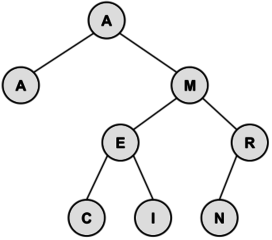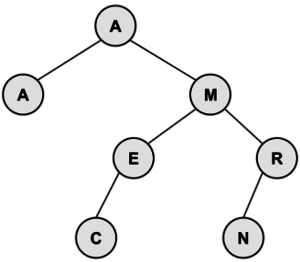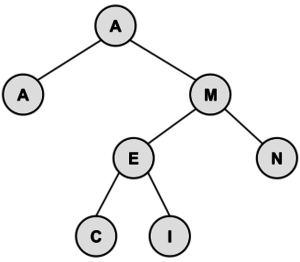### 优先队列

1. 每一个父节点都小于等于它的两个子节点（两个子节点的排放位置不受他们的大小影响）
2. 它的结构是完全二叉树——每一层都完全覆盖，除了叶节点，并且叶节点要从左到右填充

b = bottom-most and right-most element
p = root of tree
p’s key = b’s key
delete b
while (p is larger than either child)
exchange p with smaller child
p = smaller child
end while


### Questions

1. Consider an initially empty stack. After the following operations are performed, what is the value of Z?
PUSH(3)
PUSH(6)
PUSH(8)
Y = POP()
X = POP()
PUSH(X-Y)
Z = POP()

1. Create a min-heap with the letters in the word PROGRAMMING. What are the letters in the bottom-most row, from left to right?

2. Create a binary search tree from the letters in the word PROGRAM. What is the internal path length?

### Solutions

1. The first POP stores 8 in Y. The second POP stores 6 in X. Then, 6-8=-2 is pushed onto the stack. Finally, the last POP removes the -2 and stores it in Z.
2. The bottom row contains the letters RORN, from left-to-right. Here is the entire heap: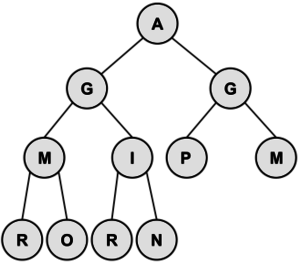3. When drawing the tree, P has a depth of 0, O and R have a depth of 1, G and R have a depth of 2, and A and M have a depth of 3. Therefore, the internal path length is 21 + 22 + 2*3 = 12. Here is the tree: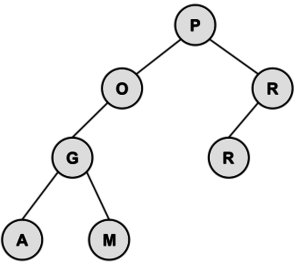## Recursive functions

def Fibonacci(x):
if x == 1 :
return 1
elif x == 2:
return 1
else:
return Fibonacci(x-1) + Fibonacci(x-2)


def Factorial(x):
if x == 0:
return 1
return x * Factorial(x-1)


### Question

1. Find g(11) given the following

$g(x)=\left\{\begin{matrix} g(x-3) + 1 \hspace{1em} if \ x > 0 \\ 3x \hspace{1em} otherwise \end{matrix}\right.$

### Solution

g(11)= g(8)+1
g(8) = g(5)+1
g(5) = g(2)+1
g(2) = g(-1)+1
g(-1)= -3

We now use what we know about g(−1) to learn more values, working our way back "up" the recursion:
We now know the value of g(2):g(2)=−3+1=−2
We now know the value of g(5):g(5)=−2+1=−1
We now know the value of g(8):g(8)=−1+1=0
And finally, we now have the value of g(11):g(11)=0+1=1

For more questions, look at this website.